Courses

# Potential Energy in an External Field and Electrostatic Shielding Class 12 Notes | EduRev

## JEE : Potential Energy in an External Field and Electrostatic Shielding Class 12 Notes | EduRev

The document Potential Energy in an External Field and Electrostatic Shielding Class 12 Notes | EduRev is a part of the JEE Course Physics Class 12.
All you need of JEE at this link: JEE

Potential energy of a dipole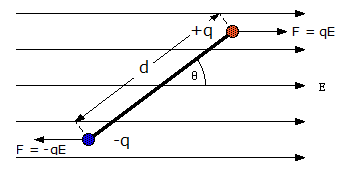Consider a dipole with charges q1 = +q and q2 = -q placed in a uniform electric field as shown in the figure above. The charges are separated by a distance d and the magnitude of electric field is E. The force experienced by the charges is given as –qE and +qE, as can be seen in the figure.
As we know that, when a dipole is placed in a uniform electric field, both the charges as a whole do not experience any force, but it experiences a torque equal to τ which can be given as,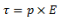This torque rotates the dipole unless it is placed parallel or anti-parallel to the field. If we apply an external and opposite torque, it neutralizes the effect of this torque given by τext and it rotates the dipole from the angle Ɵ0 to an angle Ɵ1 at an infinitesimal angular speed without any angular acceleration.
The amount of work done by the external torque can be given by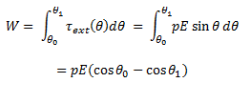As we know that the work done in bringing a system of charges from infinity to the given configuration is defined as the potential energy of the system, hence the potential energy U(Ɵ)  can be associated to the inclination Ɵ of the dipole using the above relation.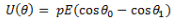From the above equation, we can see that the potential energy of dipole placed in an external field is zero when the angle Ɵ is equal to 90° or when the dipole makes an angle of 90°.
Considering the initial angle to be the angle at which the potential energy is zero, the potential energy of the system can be given as,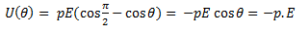Electrostatic Shielding
The third kind of shielding is a protection against electric induction, commonly called electrostatic induction. (Electrostatic induction is really a misnomer; this induction is better called electric induction, because it depends upon the continuous fluctuation of the charges induced.)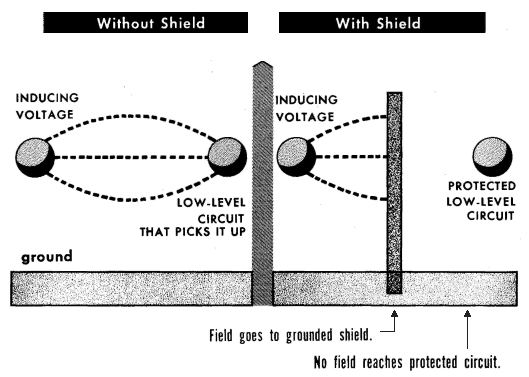Fig: Electric shielding

Electric shielding consists of interposing a grounded shield between the interfering voltage and the low-level circuit that might pick up the electric field. The voltage induced is immediately carried off to ground, and the electric field is prevented from reaching the circuit that is shielded. Unlike either form of protection against magnetic fields, shielding against electric fields can be almost 100% effective. All that is necessary is to insure that no path is left through which the electric field can pass. For this reason, much more attention is given to the prevention of induction by magnetic fields.

Offer running on EduRev: Apply code STAYHOME200 to get INR 200 off on our premium plan EduRev Infinity!

,

,

,

,

,

,

,

,

,

,

,

,

,

,

,

,

,

,

,

,

,

;